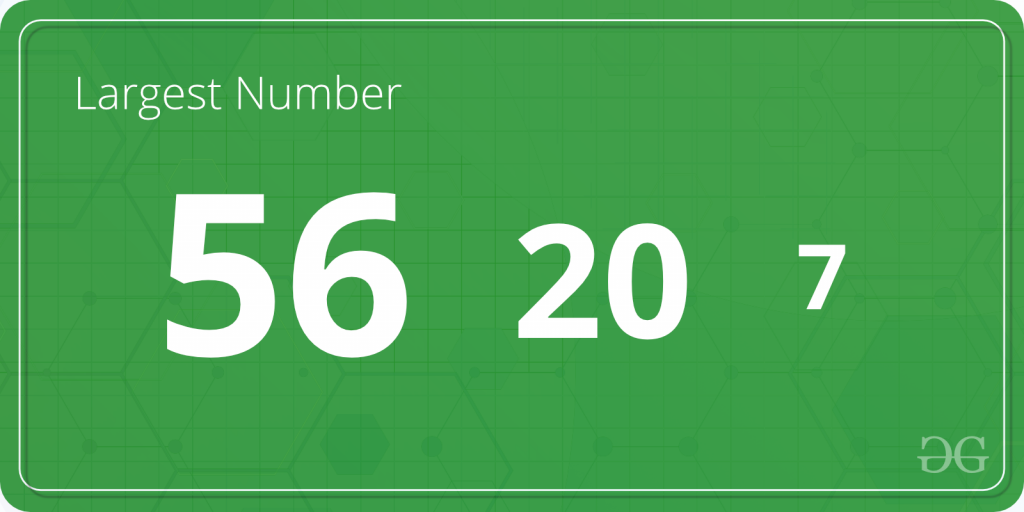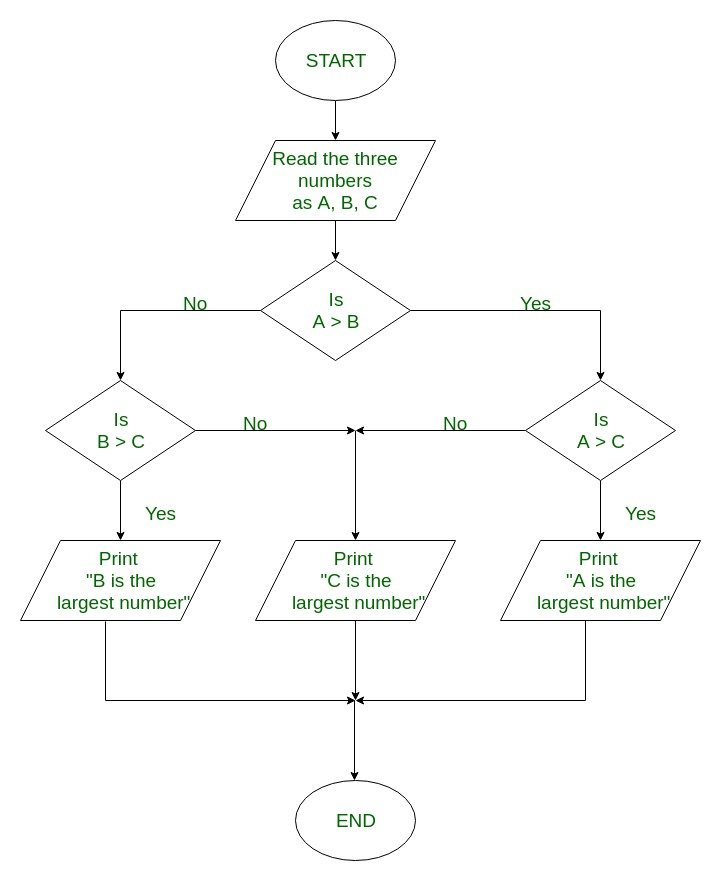GeeksforGeeks App
Open AppBrowser
Continue

# C program to Find the Largest Number Among Three Numbers

Given three numbers A, B and C; The task is to find the largest number among the three.Examples:

Input: A = 2, B = 8, C = 1
Output: Largest number = 8

Input: A = 231, B = 4751, C = 75821
Output: Largest number = 75821

## Recommended: Please try your approach on {IDE} first, before moving on to the solution.

In the below programs, to find the largest of the three number,and‘s are used. Algorithm to find the largest of three numbers:

```1. Start
2. Read the three numbers to be compared, as A, B and C.
3. Check if A is greater than B.

3.1 If true, then check if A is greater than C.
3.1.1 If true, print 'A' as the greatest number.
3.1.2 If false, print 'C' as the greatest number.

3.2 If false, then check if B is greater than C.
3.1.1 If true, print 'B' as the greatest number.
3.1.2 If false, print 'C' as the greatest number.
4. End```

FlowChart to find the largest of three numbers:Below is the C program to find the largest among the three numbers: Example 1: Using only if statements to find the largest number.

## C

 `#include  `` ` `int` `main() ``{ ``    ``int` `A, B, C; `` ` `    ``printf``(``"Enter the numbers A, B and C: "``); ``    ``scanf``(``"%d %d %d"``, &A, &B, &C); `` ` `    ``if` `(A >= B && A >= C) ``        ``printf``(``"%d is the largest number."``, A); `` ` `    ``if` `(B >= A && B >= C) ``        ``printf``(``"%d is the largest number."``, B); `` ` `    ``if` `(C >= A && C >= B) ``        ``printf``(``"%d is the largest number."``, C); `` ` `    ``return` `0; ``} `

Output:

```Enter the numbers A, B and C: 2 8 1
8 is the largest number.```

Time complexity: O(1) as it is doing constant operations
Auxiliary space: O(1)

Example 2: Using if-else statement to find the largest number.

## C

 `#include  ``int` `main() ``{ ``    ``int` `A, B, C; `` ` `    ``printf``(``"Enter three numbers: "``); ``    ``scanf``(``"%d %d %d"``, &A, &B, &C); `` ` `    ``if` `(A >= B) { ``        ``if` `(A >= C) ``            ``printf``(``"%d is the largest number."``, A); ``        ``else``            ``printf``(``"%d is the largest number."``, C); ``    ``} ``    ``else` `{ ``        ``if` `(B >= C) ``            ``printf``(``"%d is the largest number."``, B); ``        ``else``            ``printf``(``"%d is the largest number."``, C); ``    ``} `` ` `    ``return` `0; ``} `

Output:

```Enter the numbers A, B and C: 2 8 1
8 is the largest number.```

Time complexity: O(1) as it is doing constant operations
Auxiliary space: O(1)

Example 3: Using nested if-else statements to find the largest number.

## C

 `#include  ``int` `main() ``{ ``    ``int` `A, B, C; `` ` `    ``printf``(``"Enter three numbers: "``); ``    ``scanf``(``"%d %d %d"``, &A, &B, &C); `` ` `    ``if` `(A >= B && A >= C) ``        ``printf``(``"%d is the largest number."``, A); `` ` `    ``else` `if` `(B >= A && B >= C) ``        ``printf``(``"%d is the largest number."``, B); `` ` `    ``else``        ``printf``(``"%d is the largest number."``, C); `` ` `    ``return` `0; ``} `

Output:

```Enter the numbers A, B and C: 2 8 1
8 is the largest number.```

Time complexity: O(1) as it is doing constant operations
Auxiliary space: O(1)

Example 4: Using Ternary operator to find the largest number.

## C

 `#include  ``int` `main() ``{ ``    ``int` `A, B, C, largest; `` ` `    ``printf``(``"Enter three numbers: "``); ``    ``scanf``(``"%d %d %d"``, &A, &B, &C); `` ` `    ``largest = A > B ? (A > C ? A : C) : (B > C ? B : C); `` ` `    ``printf``(``"%d is the largest number."``, largest); `` ` `    ``return` `0; ``} `

Output:

```Enter the numbers A, B and C: 2 8 1
8 is the largest number.```

Time complexity: O(1) as it is doing constant operations
Auxiliary space: O(1)

My Personal Notes arrow_drop_up